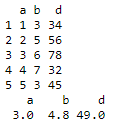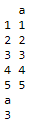Open in App
Not now

# Calculate mean of multiple columns of R DataFrame

• Last Updated : 09 May, 2021

Mean is a numerical representation of the central tendency of the sample in consideration. In this article, we are going to calculate the mean of multiple columns of a dataframe in R Programming Language.

Formula:

Mean= sum of observations/total number of observations.

### Method 1: Using colMeans() function

colMeans() this will return the column-wise mean of the given dataframe.

Syntax:

colMeans(dataframe_name)

where dataframe_name is the input dataframe.

For this simply pass the dataframe in use to the colMeans() function. The result will be the mean of all the individual columns.

Example:

## R

 `# create a vector 1 ` `a=``c``(1,2,3,4,5) ` ` `  `# create a vector 2 ` `b=``c``(3,5,6,7,3) ` ` `  `# create a vector 3 ` `d=``c``(34,56,78,32,45) ` ` `  `# pass these vectors to  ` `# dataframe ` `data=``data.frame``(a,b,d) ` ` `  `print``(data) ` ` `  `# mean columns of the  ` `# dataframe ` `print``(``colMeans``(data)) `

Output:Example 2:

## R

 `# create a vector 1 ` `a=``c``(1,2,3,4,5) ` ` `  `# pass the vector to data frame ` `data=``data.frame``(a) ` ` `  `print``(data) ` ` `  `# mean column of the dataframe ` `print``(``colMeans``(data)) `

Output:### Method 2: Using sapply() function

Syntax:

sapply(dataframe,mean)

where dataframe is the input dataframe and mean is the method to calculate mean.

For this dataframe name needs to be passed along with the action to perform in our case mean.

Example:

## R

 `# numeric vector a ` `a=``c``(1,2,3,4,5) ` ` `  `# numeric vector b ` `b=``c``(3,4,5,6,7) ` ` `  `# numeric vector d ` `d=``c``(34.6,78.8,89,9.43,67.9) ` ` `  `# pass the vectors to data frame ` `data=``data.frame``(a,b,d) ` ` `  `# use sapply function to calculate mean ` `print``(``sapply``(data,mean)) `

Output:

```     a      b      d
3.000  5.000 55.946 ```

Example 2

## R

 `# numeric vector a ` `a=``c``(1,2,3,4,5) ` ` `  `# pass the vector to data frame ` `data=``data.frame``(a) ` ` `  `# use sapply function to calculate mean ` `print``(``sapply``(data,mean))`

Output:

```a
3```

My Personal Notes arrow_drop_up
Related Articles